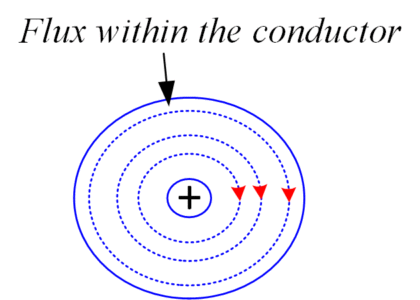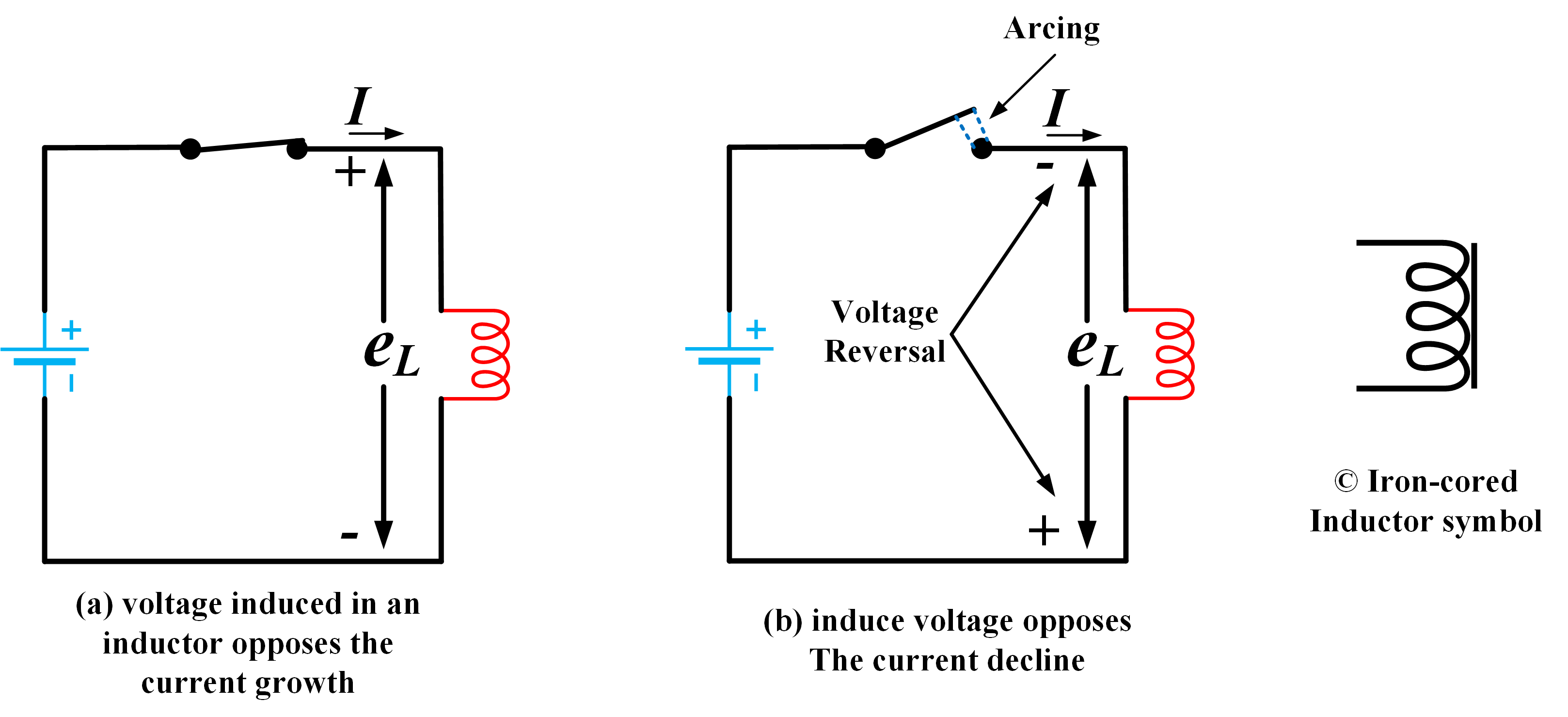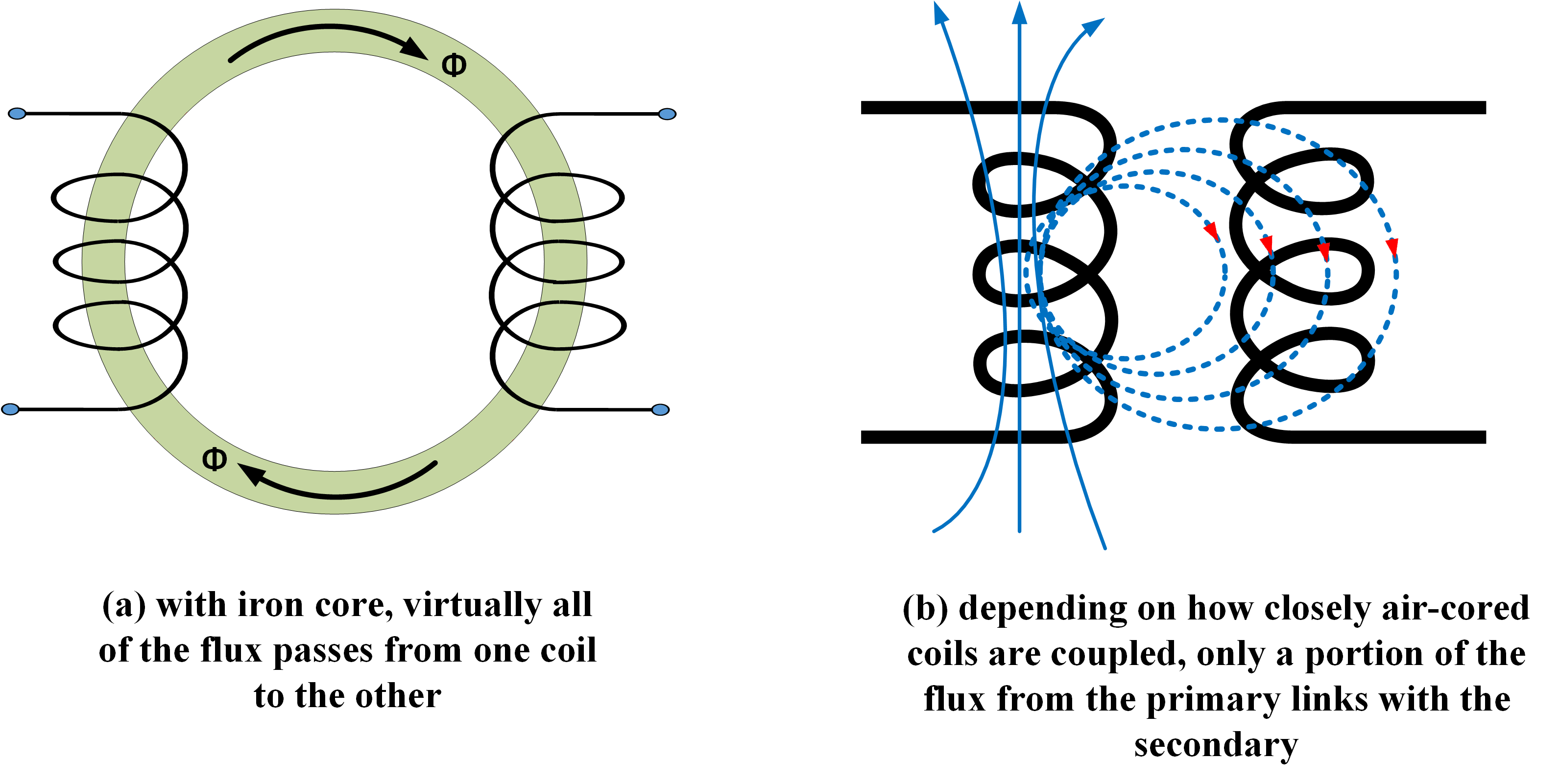Home / Basic Electrical / Mutual Inductance and Self Inductance | Formula & Example

# Mutual Inductance and Self Inductance | Formula & Example

Want create site? Find Free WordPress Themes and plugins.

Electromagnetic induction occurs when a magnetic flux in motion with respect to a single conductor or a coil induces an emf in the conductor or coil. Because the growth or decline of current through a coil generates a changing flux, an emf is induced in the coil by its own current change. The same effect can induce an emf in an adjacent coil. The level of emf induced in each case depends on the self-inductance of the coil, or on the mutual inductance between the two coils. In all cases, the polarity of the induced emf is such that it opposes the original change that induced the emf.

Components called inductors or chokes are constructed to have specified values of inductance. Inductors can be operated in series or in parallel. Even the shortest of conductors has an inductance. This is usually an unwanted quantity and is termed stray inductance.

## Coil and Conductor Inductance

It has been shown that an emf is induced in a conductor moving through a magnetic ﬁeld and that the growth of current in a coil can induce an emf in another magnetically coupled coil. It is also possible for a coil to induce a voltage in itself as its current level changes. This phenomenon is known as self-inductance, and the principle is illustrated in Figure 1.Fig.1: Current Carrying Coil and its Cross-Sectional Area

Magnetic flux growing outwards around the turns of a coil cuts (or brushes over) the other coil turns and induces emf in the coil

A coil and its cross-sectional area are shown in Figure 1, with arrow tails and points indicating the current directions in each turn. Every turn of the coil has a flux around it produced by the current ﬂowing through the coil. However, for convenience, the illustration shows the growth of flux around only one turn on the coil. It is seen that as the current grows, the flux expands outward and cuts (or brushes over) the other turns. This causes currents to be induced in the other turns, and the direction of the induced currents is such that they set up a ﬂux that opposes the ﬂux inducing them.

Remembering that the current through the coil causes the ﬂux to grow around all turns at once, it is seen that the ﬂux from every turn induces a current that opposes it in every other turn.

To set up opposing ﬂuxes, the induced current in a coil must be in opposition to the current ﬂowing through the coil from the external source of supply. The induced current is, of course, the result of an induced emf. Thus, it is seen that the self-inductance of a coil sets up an induced emf that opposes the external emf that is driving current through the coil. Because this induced emf is in opposition to the supply voltage, it is usually termed the counter-emf or back-emf. The counter-emf occurs only when the coil current is growing or declining. When the current has reached a constant level, the ﬂux is no longer changing and no counter-emf is generated.

Even a single conductor has self-inductance. Figure 2 shows that when current is growing in a conductor, ﬂux may grow outward from the center of the conductor. This ﬂux cuts other portions of the conductor and induces a counter-emf.Fig.2: conductor cross section

The growth of current within a conductor induces emfs in other portions of the conductor.

In Figure 3, the polarity of the counter-emf induced in a coil is illustrated for a given supply voltage polarity. In Figure 3(a), the switch is closed and current I commences to grow from zero. The polarity of the counter-emf (eL) is such that it opposes the growth of I, thus it is series-opposing with the supply voltage. When the switch is opened (figure 3(b)), the current tends to fall to zero. But now the polarity of eL is such that it opposes the decline of I. it is series-aiding with the supply voltage. In fact, eL may cause arcing at the switch terminals as it depends on the coil’s inductance.Fig.3: Induced emf polarity

The counter-emf induced in a coil always opposes the growth or decline of the current.

The SI unit of inductance is the Henry (H).

The inductance of a circuit is one Henry when an emf of 1 V is induced by the current changing at the rate of 1 A/s.

Thus the relationship among inductance, induced voltage, and rate of change of current is:

$\begin{matrix} L=\frac{{{e}_{L}}}{{\Delta i}/{\Delta t}\;} & {} & \left( 1 \right) \\\end{matrix}$

Where L is the inductance in Henry, eL is the induced counter-emf in volts and is the rate of change of current in A/s. a negative sign is sometimes included in front of eL to show that the induced emf is in opposition to the applied emf. When eL=1V, and =1A/s, L=1H. If the rate of change of current is 2 A/s and eL=1V, the inductance is 0.5 H.

A coil constructed to have a certain inductance is usually referred to as an inductor or choke. Note the graphic symbols for an inductor shown in figure 3.

## Self-Inductance Formula

An expression for inductance can be derived involving the coil dimensions and the number of turns [see figure 4].Fig.4: Number of turns in a coil

The inductance of a coil depends on the number of turns and on the flux and current changes.

From equation (2):

$\begin{matrix} {{e}_{L}}=N\frac{\Delta \phi }{\Delta t} & {} & \left( 2 \right) \\\end{matrix}$

Substituting for eL into equation (1) gives

$L=N\frac{{\Delta \phi }/{\Delta t}\;}{{\Delta i}/{\Delta t}\;}$

Or

$\begin{matrix} L=N\frac{\Delta \phi }{\Delta i} & {} & \left( 3 \right) \\\end{matrix}$

Also,

$\phi =B\times A$

And

$B={{\mu }_{o}}\times {{\mu }_{r}}\times H={{\mu }_{o}}\times {{\mu }_{r}}\times \frac{IN}{l}$

Therefore,

$\phi ={{\mu }_{o}}\times {{\mu }_{r}}\times IN\times \frac{A}{l}$

Since I is a maximum current level, it also represents the change in current (∆i) from zero to the maximum level. Therefore, change in flux is

$\Delta \phi ={{\mu }_{o}}\times {{\mu }_{r}}\times \Delta i\times N\times \frac{A}{l}$

Substituting for ∆ϕ in equation (3) gives

$L=\frac{\left( {{\mu }_{o}}\times {{\mu }_{r}}\times \Delta i\times N\times {}^{A}/{}_{l} \right)\times N}{\Delta i}$

Or

$\begin{matrix} L={{\mu }_{o}}\times {{\mu }_{r}}\times {{N}^{2}}\times {}^{A}/{}_{l} & {} & \left( 4 \right) \\\end{matrix}$

Note that, as illustrated in Figure 5, the inductance is proportional to the cross-sectional area of a coil and to the square of the number of turns. It is also inversely proportional to the coil length. Therefore, maximum inductance is obtained with a short coil that has a large cross-sectional area and a large number of turns.Fig.5: Coil Dimensions

Coil inductance can be calculated from its dimensions and its core permeability.

Equation (4) now affords a means of calculating the inductance of a coil of known dimensions. Alternatively, it can be used to determine the required dimensions for a coil to have a given inductance. However, it is not so easily applied to iron-cored coils, because the permeability of ferromagnetic material changes when the ﬂux density changes. Consequently, the inductance of an iron-cored coil is constantly changing as the coil current increases and decreases.

## Non-inductive Coil

In many cases it is desired to have a non-inductive coil; for example, precision resistors are usually non-inductive. To construct such a coil, the winding is made of two side-by-side conductors, as illustrated in Figure 6. Every coil turn has an adjacent turn carrying current in the Opposite direction. The magnetic ﬁelds generated by adjacent turns cancel each other out. Therefore, no counter-emf is generated, and the coil is non-inductive.Fig.6: Non-Inductive Coil

## Self-Inductance Example

A solenoid with 900 turns has a total ﬂux of 1.33 X 10-7 Wb through its air core when the coil current is 100 mA. If the ﬂux takes 75 ms to grow from zero to its maximum level, calculate the inductance of the coil. Also, determine the counter-emf induced in the coil during the ﬂux growth.

Solution

\begin{align} & \Delta \phi =1.33\times {{10}^{-7}}Wb \\ & \Delta i=100mA \\ & \Delta t=75ms \\\end{align}

Equation (3):

$L=N\frac{\Delta \phi }{\Delta i}=900\frac{1.33\times {{10}^{-7}}}{100\times {{10}^{-3}}}=1.2mH$

From equation (2)

${{e}_{L}}=N\frac{\Delta \phi }{\Delta t}=900\frac{1.33\times {{10}^{-7}}}{75\times {{10}^{-3}}}=1.6mV$

## Mutual Inductance

When the ﬂux from one coil cuts another adjacent (or magnetically coupled) coil, an emf is induced in the second coil. Following Lenz’s law, the emf induced in the second coil sets up a ﬂux that opposes the original ﬂux from the ﬁrst coil. Thus, the induced emf is again a counter-emf, and in this case the inductive effect is referred to as mutual inductance. Figure 7 shows the graphic symbols used for coils with mutual inductance, also termed coupled coils.Fig.7: Graphic symbols for Air and Iron Cored Coils

Like self-inductance, mutual inductance is measured in Henry (H).

## Mutual Inductance Formula

Two coils have a mutual inductance of 1H when an emf of 1V is induced in one coil by current changing at the rate of 1 A/s in the other coil.

This definition gives rise to the equation relating mutual inductance to induced voltage and rate of change of current:

$\begin{matrix} M=\frac{{{e}_{L}}}{{\Delta i}/{\Delta t}\;} & {} & \left( 5 \right) \\\end{matrix}$

Where M is the mutual inductance in Henry, eL is the emf in volts induced in the secondary coil and is the rate of change of current in the primary coil in A/s.

The coil through which a current is passed from an external source is termed the primary, and the coil that has an emf induced in it is referred to as the secondary.

An equation for the emf induced in the secondary coil can be written as:

$\begin{matrix} {{e}_{L}}={{N}_{s}}\frac{\Delta \phi }{\Delta t} & {} & \left( 6 \right) \\\end{matrix}$

Here ∆ϕ is the total change in flux linking with the secondary winding, Ns is the number of turns in the secondary winding, and ∆t is the time required for the flux change.

Substituting for eL from equation (6) into equation (5) gives

$M={{N}_{s}}\frac{{\Delta \phi }/{\Delta t}\;}{{\Delta i}/{\Delta t}\;}$

Therefore,

$\begin{matrix} M={{N}_{s}}\frac{\Delta \phi }{\Delta i} & {} & \left( 7 \right) \\\end{matrix}$

Figure 8(a) illustrates the fact that when the two coils are wound on a single ferromagnetic core, effectively all of the flux generated by the primary coil links with the secondary coil. However, when the coils are air-cored, only a portion of the flux from the primary may link with the secondary [see figure 8 (b)]. Depending on how much of the primary ﬂux cuts the secondary, the coils may be classiﬁed as loosely coupled or tightly coupled. One way to ensure tight coupling is shown in Figure 8(c), where each turn of the secondary winding is side by side with one turn of the primary winding. Coils wound in this fashion are said to bifilar.Fig.8: Flux linkages in primary and secondary coils

The amount of flux from a primary winding that links with a secondary depends on how closely the coils are coupled. The coefficient of coupling defines the linkage.

The amount of flux linking from primary to secondary is also defined in terms of a coefficient of coupling, k. If all the primary ﬂux links with the secondary, the coefﬁcient of coupling is 1. When only 50% of the primary ﬂux links with the secondary coil, the coefﬁcient of coupling is 0.5. Thus,

$k=\frac{flux\text{ }linkages\text{ }between\text{ }primary\text{ }and\text{ }\sec ondary}{total\text{ }fluxproduced\text{ }by\text{ }primary}$

Returning to equation (7). When ∆ϕ is the total flux change in the primary coil, the flux linking with the secondary is k∆ϕ. Therefore, the equation for M

$\begin{matrix} M=k{{N}_{s}}\frac{\Delta \phi }{\Delta i} & {} & \left( 8 \right) \\\end{matrix}$

Also, substituting for $\Delta \phi ={{\mu }_{o}}\times {{\mu }_{r}}\times \Delta i\times N\times \frac{A}{l}$ into equation (8) gives

$M=\frac{k{{N}_{s}}}{\Delta i}\times{{\mu }_{o}}\times {{\mu }_{r}}\times \Delta i\times {{N}_{p}}\times \frac{A}{l}$

Or

$\begin{matrix} M=k\times {{N}_{p}}\times {{N}_{s}}\times {{\mu }_{o}}\times {{\mu }_{r}}\times \frac{A}{l} & {} & \left( 9 \right) \\\end{matrix}$

Each winding considered alone has a self-inductance that can be calculated from equation (4). Thus, for the primary coil,

${{L}_{1}}=N_{p}^{2}\times {{\mu }_{o}}\times {{\mu }_{r}}\times \frac{A}{l}$

And for the secondary

${{L}_{2}}=N_{s}^{2}\times {{\mu }_{o}}\times {{\mu }_{r}}\times \frac{A}{l}$

Assuming that the two windings share the common core (magnetic or non-magnetic as in figure 9), the only difference in the expression for L1 and L2 is the number of turns.Fig.9: Two windings on the same core

Therefore,

${{L}_{1}}\times {{L}_{2}}=N_{p}^{2}\times N_{p}^{2}\times {{\left( {{\mu }_{o}}\times {{\mu }_{r}}\times \frac{A}{l} \right)}^{2}}$

Or

$\begin{matrix} \sqrt{{{L}_{1}}\times {{L}_{2}}}={{N}_{p}}\times {{N}_{s}}\times {{\mu }_{o}}\times {{\mu }_{r}}\times \frac{A}{l} & {} & \left( 10 \right) \\\end{matrix}$

Comparing equations 9 and 10, it is seen that,

$\begin{matrix} M=k\sqrt{{{L}_{1}}\times {{L}_{2}}} & {} & \left( 11 \right) \\\end{matrix}$

## Mutual Inductance Example

Two identical coils are wound on a ring-shaped iron core that has a relative permeability of 500. Each coil has 100 turns, and the core dimensions are: cross-sectional area A=3 cm2 and magnetic path length l=20cm. calculate the inductance of each coil and the mutual inductance between the coils.

Solution

From equation (4):

\begin{align} & {{L}_{1}}={{L}_{2}}={{N}^{2}}\times {{\mu }_{o}}\times {{\mu }_{r}}\times \frac{A}{l} \\ & ={{100}^{2}}\times 500\times 4\pi \times {{10}^{-7}}\times \frac{3\times {{10}^{-4}}}{20\times {{10}^{-2}}}\cong 9.42mH \\\end{align}

As the coils are wound on the same iron core, k=1. Equation (11):

$M=k\sqrt{{{L}_{1}}\times {{L}_{2}}}=\sqrt{9.42\times 9.42}=9.42mH$

Did you find apk for android? You can find new Free Android Games and apps.

### About Ahmad FaizanMr. Ahmed Faizan Sheikh, M.Sc. (USA), Research Fellow (USA), a member of IEEE & CIGRE, is a Fulbright Alumnus and earned his Master’s Degree in Electrical and Power Engineering from Kansas State University, USA.